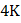# A ball of massmoving with velocitymakes an oblique elastic collision with a stationary ball of mass 2The angle of divergence between the balls after  collision in ground frame, if the ball of massturns by an angle ofin centre of mass frame is a)b)c)d)## Question ID - 100211 :- A ball of massmoving with velocitymakes an oblique elastic collision with a stationary ball of mass 2The angle of divergence between the balls after  collision in ground frame, if the ball of massturns by an angle ofin centre of mass frame is a)b)c)d)3537

(d)

Let us solve this question inframe. As the collision is elastic, the magnitude of momenta of all balls remains same after collision in this frame

Velocity offrame w.r.t. ground frame isThe momentum diagram inframe is drawn as shown in fig.Now, velocity ofafter colision inframe iswhile that ofisalongand,  respectively. Letandbe the velocities ofand, respectively after collision in ground frame, then velocity vector diagrams can be drawn as shownUsing trigonometry, from upper triangleFrom lower triangle:So, angle of divergence isAlternative method  Write down conservation of momentum equations, coefficient of restitution equation, energy conservation and use the given conditions

Next Question :
 A child is standing with folded hands at the centre of a platform rotating about its central axis. The kinetic energy of the system is. The child stretches his arms so that the moment of inertia of the system doubles. The kinetic energy of the system now is a)b)c)d)